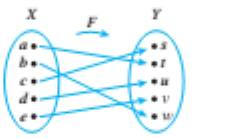Chapter 7.2, Problem 43ES### Discrete Mathematics With Applicat...

5th Edition
EPP + 1 other
ISBN: 9781337694193

#### Solutions

Chapter
Section### Discrete Mathematics With Applicat...

5th Edition
EPP + 1 other
ISBN: 9781337694193
Textbook Problem
1 views

# Let X = { a , b , c , d , e } and Y = { s , t u , v , w } . In each of 42 and 43 a one-to-one corrspondence F : X → Y is defined by an arrow diagram. In each case draw an arrow diagrm for F − 1 .To determine

To draw:

An arrow diagram for F1.

Explanation

Given information:

Arrow diagram of F is as follows:

Concept used:

A function F is said to be one-to-one function if, and only if, the distinct elements in its domain are mapped with the distinct elements in its co-domain.

A function is said to be onto function if, and only if, each element in its codomain is the image of at least one element in its domain.

Calculation:

F is given by the arrow diagram.

Clearly, from the given figure, F is one-to-one and onto function.

So, its inverse function exists

### Still sussing out bartleby?

Check out a sample textbook solution.

See a sample solution

#### The Solution to Your Study Problems

Bartleby provides explanations to thousands of textbook problems written by our experts, many with advanced degrees!

Get Started